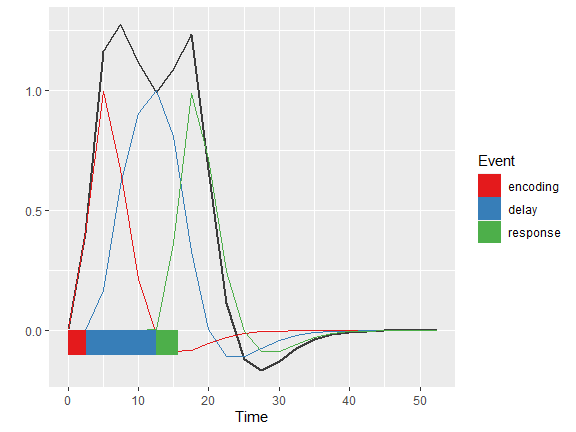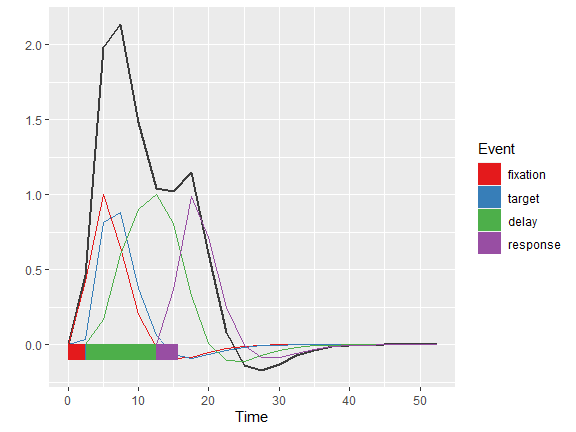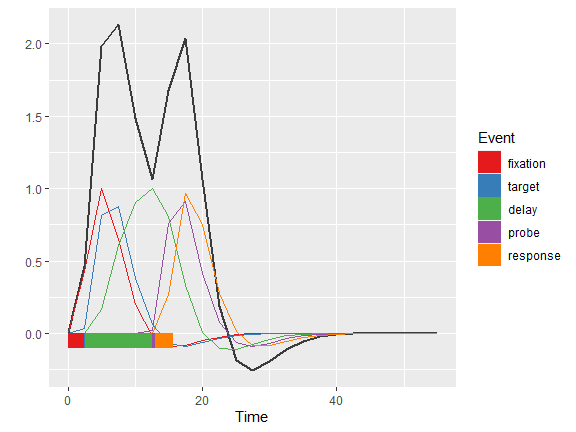# An example about how to evaluate manually constructed models with the autohrf package

#### 2022-09-30

The autohrf package is not only useful for preparing model specifications and then automatically finding the models that best fit the underlying data. In this example we will show how you can use the autohrf package to investigate the quality of manually constructed models.

Let us start this example by loading required libraries and the data from the spatial working memory experiment (SWM).

# libs
library(autohrf)

# load the data
df <- swm
head(df)
##         roi t        y
## 1   L_2_ROI 0 2.445844
## 2  L_46_ROI 0 2.155406
## 3  L_6a_ROI 0 4.436729
## 4 L_7Am_ROI 0 1.169667
## 5 L_AIP_ROI 0 4.132354
## 6 L_FEF_ROI 0 7.725104

The loaded data frame has 504 observations, each with 3 variables (roi, t, and y) roi denotes the region of interest, t the time stamp and y the value of the BOLD signal. Note that input data for the autohrf package should be always organized in this manner.

Next, we will construct three different models, one with 3 events, one with 4 events and one with 5 events. When manually constructing events we need to create a data frame which has an entry (observation) for each of the events in the model. For each of the events we need to provide its name, its start time and its duration.

# 3 events: encoding, delay, response
model3 <- data.frame(event      = c("encoding", "delay", "response"),
start_time = c(0,           2.65,    12.5),
duration   = c(2.65,        9.85,    3))

# 4 events: fixation, target, delay, response
model4 <- data.frame(event      = c("fixation", "target", "delay", "response"),
start_time = c(0,           2.5,      2.65,    12.5),
duration   = c(2.5,         0.1,      9.85,    3))

# 5 events: fixation, target, delay, probe, response
model5 <- data.frame(event      = c("fixation", "target", "delay", "probe", "response"),
start_time = c(0,           2.5,      2.65,    12.5,    13),
duration   = c(2.5,         0.1,      9.85,    0.5,     2.5))

Once we construct our models we can use the convolve_events function to convolve the HRF signal based on the model with the actual data. Once we have that, we can use the run_model function to evaluate which model fits our data best. We can also use the plot_model function to visually inspect how model fits the underlying data.

# 3 events
em3 <- evaluate_model(df, model3, tr = 2.5)
##
## Mean R2:  0.9260475
## Median R2:  0.9458051
## Min R2:  0.8115323
## Weighted R2:  0.9260475
##
## Mean BIC:  102.7654
## Median BIC:  102.5578
## Min BIC:  60.79281
## Weighted BIC:  102.7654
plot_model(em3)# 4 events
em4 <- evaluate_model(df, model4, tr = 2.5)
##
## Mean R2:  0.9444968
## Median R2:  0.9654673
## Min R2:  0.8393465
## Weighted R2:  0.9444968
##
## Mean BIC:  102.0323
## Median BIC:  102.1207
## Min BIC:  58.60426
## Weighted BIC:  102.0323
plot_model(em4)em5 <- evaluate_model(df, model5, tr = 2.5)
##
## Mean R2:  0.9728839
## Median R2:  0.9884729
## Min R2:  0.8553543
## Weighted R2:  0.9728839
##
## Mean BIC:  91.80148
## Median BIC:  88.81185
## Min BIC:  60.47441
## Weighted BIC:  91.80148
plot_model(em5)We can see that the best R2 score was achieved by our model with 5 events. So it would make sense to use that one for further assumed GLM modelling.